# Standard Form of Numbers, Fractions, and Decimals

### An introduction to Standard Form of Numbers, Fractions, and Decimals: Explained with Examples

In mathematics, a standard form is a way of representing numbers, fractions, and decimals in a standard format that is easy to read and write. It has wide uses in science and engineering for various purposes.

In this article, we will explain the standard form of numbers, fractions, and decimals with steps and solved examples.

#### What is the standard form of numbers?

In number theory, a standard way to write numbers in a single digit before the decimal point along with the exponent of 10 is said to be the standard form of numbers. The digit before the decimal point could be 1, 2, 3, 4, 5, 6, 7, 8, or 9.

For example, the number 345670000 can be written in the standard form as 3.4567 x 108. The way of representing a very larger number or very small number in standard notation is said to be the standard form of a number.

#### Steps to write numbers in standard form

Here are a few steps to write numbers in standard form.

 Steps Example First of all, take a number. 1230000000000 Put the decimal point after the first significant digit (single digit before the decimal point). = 1.230000000000 Count the number of digits after the significant digit and write it in the power of 10. Digits after decimal point = 12 1012 Multiply the significant digits with the power of 10 and ignore the proceeding zeros. 1.23 x 1012

#### Examples of writing numbers in standard form

Here are a few examples to write the number in standard form .

Example 1

Convert 65700000 in the standard form.

Solution

Step 1: Write the decimal point after the first significant digit.

First significant digit = 6

6.5700000

Step 2: Now count the digits after the decimal point and write it in the power of 10.

6.5700000 x 107

Step 3: Ignore the zeros after the decimal point.

6.57x 107

Hence, 6.57x 107 is the standard notation of 65700000

Example 2

Convert 0. 0000000347 in the standard form.

Solution

Step 1: Write the decimal point after the first significant digit.

First significant digit = 3

000000003.47

Step 2: Now count the digits before the decimal point and write it in the power of 10 with a negative sign.

000000003.47 x 10-8

Step 3: Ignore the zeros before the decimal point.

3.47 x 10-8

Hence, 3.47 x 10-8 is the standard notation of 000000003.47

#### What is the standard form of fractions?

In algebra, a way of writing fractions in a standard notation by converting them into decimals is said to be the standard form of fractions.

The digits before the decimal could be any significant digit. The standard form of fractions can also be written as the fraction is divided with a suitable integer to the numerator and denominator to make them co-prime.

For example, 4/6 can be written in standard form as 2/3. Alternatively, the 4/6 in decimal is 0.667 and its standard form is 6.67 x 10-1.

#### Steps to write fractions in standard form

Here are a few steps to write fractions in standard form.

Case 1

 Steps Example First of all, take a fraction. 24/36 Convert the fraction into a decimal number by dividing the numerator by the denominator. 24/36 = 0.667 Put the decimal point after the first significant digit 06.67 Count the number of digits before the significant digit and write it in the power of 10 with a negative sign. 06.67 x 10-1 Ignore the leading zeros. 6.67 x 10-1

Case 2:

 Steps Example First of all, take the fraction 40/36 Divide the numerator and denominator with a suitable multiplication table. 40 ÷ 4 / 36 ÷ 4 Repeat step 2 until the numerator and denominator become co-prime. 10/9

#### Examples of writing fractions in standard form

Here are a few examples of writing fractions in standard notation.

Example 1

Convert 124/76 in the standard form.

Solution

Step 1: Convert the fraction into a decimal number by dividing the numerator by the denominator.

Step 2: Now write the given result and multiply it with the power of 10.

1.6316 x 100

Hence, 1.6316 x 100 is the standard notation of 124/76

Example 2

Convert 224/76 in the standard form.

Solution

Step 1: Divide the numerator and denominator of the given fraction by 2

224 ÷ 2 /76 ÷ 2

= 112/38

Step 2: The numerator and denominator are still not co-prime, divide them by 2 again.

112 ÷ 2 /38 ÷ 2

= 56/19

Hence, 56/19 are co-prime so it is the standard notation of 224/76

#### What is the standard form of decimals?

In mathematics, the way of representing the decimal number after the first significant digit to any decimal number is said to be the standard form of decimals. There are two possible ways to write any decimal number in the standard notation.

• If the decimal point moves from left to right, then the power of 10 will be negative.
• If the decimal point moves from right to left, then the power of 10 will be positive.

#### Steps to write fractions in standard form

Here are a few steps to write a decimal in standard form.

Case 1:Decimal point move from right to left

 Steps Example First of all, take a decimal number. 243.64 Move the decimal point from right to left after the first significant digit.. 243.64 → 2.4364 Count the number of digits between the significant digit and the original decimal place. Then write counted digit of the above number in the power of 10 with a positive sign. 2.4364 x 102

Case 2:Decimal point move from left to right

 Steps Example First of all, take a decimal number. 0.00002404 Move the decimal point from left to right after the first significant digit. 0.00002404 → 000002.404 Count the number of digits between the significant digit and the original decimal place. Then write counted digit of the above number in the power of 10 with a negative sign. 000002.404 x 10-5 Ignore the leading zeros 2.404 x 10-5

#### Examples of writing decimal numbers in standard form

Here are a few examples of writing decimal numbers in standard notation.

Example 1

Convert 12345.56 in the standard form.

Solution

Step 1: First of all, Change the decimal point from right to left after the first significant digit.

First significant digit = 1

1.234556

Step 2: Now Count the number of digits between the significant digit and the original decimal place. Then write counted digit of the above number in the power of 10 with a positive sign.

Counted digits = 4

1.234556 x 104

Hence, 1.234556 x 104 is the standard notation of 12345.56

Example 2

Convert 0.000012 in the standard form.

Solution

Step 1: First of all, Change the decimal point from left to right after the first significant digit.

First significant digit = 1

000001.2

Step 2: Now Count the number of digits between the significant digit and the original decimal place. Then write counted digit of the above number in the power of 10 with a negative sign.

Counted digits = 5

000001.2 x 10-5

Step 3: Ignore the zeros before the decimal point.

1.2 x 10-5

Hence, 1.2 x 10-5 is the standard notation of 0.000012

#### Table of writing numbers fractions, and decimals in standard form

Below is a table of writing numbers fractions, and decimals in standard form

 Number, Fraction, or Decimal Standard form 10 1 * 10^1 0.000045 4.5 * 10^-5 4300000 4.3 * 10^6 0.025 2.5 * 10^-2 2/3 6.6667 * 10^-1 0.000006789 6.789 * 10^-6 0.0000003 3 * 10^-7 1000 1 * 10^3 0.001 1 * 10^-3 0.000025 2.5 * 10^-5 3/4 7.5 * 10^-1 5/8 7.8125 * 10^-1 0.05 5 * 10^-2 0.0075 7.5 * 10^-3 4345.2 4.3452 * 10^3 87890 8.789 * 10^4 1/2 5 * 10^-1

#### Conclusion

In mathematics, the standard form of numbers, fractions, and decimals provides a uniform and standardized way of expressing them. Following the above steps and examples will help you write them correctly in standard form.

Click on the following image link to check out math times table quiz.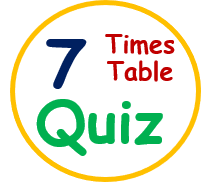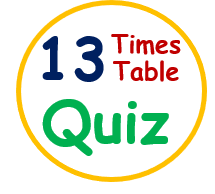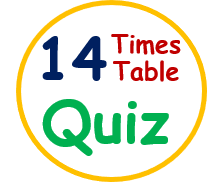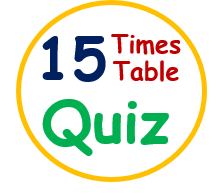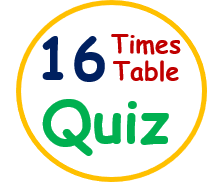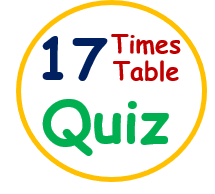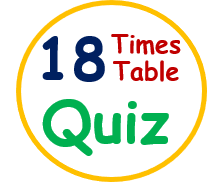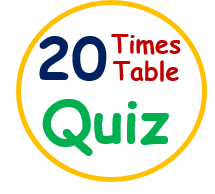If you are looking for some other topics of General knowledge like Animal GK, Solar System GK, Interesting facts about animals and birds, Science GK, Computer GK, Logical maths riddles, General Riddles, World’s Top 10, Sports GK, Inventors, and their invention, Please do visit our YouTube channel by clicking on Shiv Kids Education.

After clicking on the above URL, You will reach to our YouTube channel named “Shiv Kids Education” with a Home page that contains recently added videos. You can access all videos by clicking on the “VIDEOS” tab over there and can also view playlist videos by hitting the “PLAYLIST” tab option.

Enjoy here other topics as well by clicking on the below-given links, We ensure that you would have a wonderful GK Quizzing!